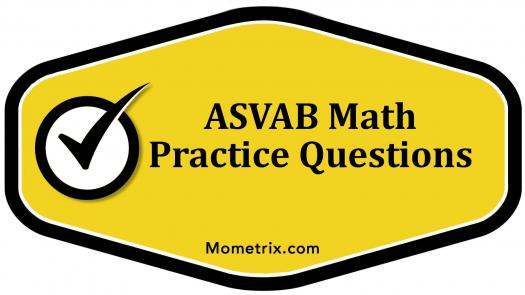# ASVAB Practice Math Questions

10 Questions | Total Attempts: 159SettingsASVAB Mathematics is test organised by the above named organization to check the weakness of candidates or applicants in the area of mathematics. The quiz will be of help in attempting the ASVAB mathematics questions which is of high passing score and required of candidates' success. Are you an ASVAB Math applicant? Try and take this easy one!

• 1.
What is the product of 3 and 5?
• A.

2

• B.

10

• C.

8

• D.

15

• 2.
What is the mode of these numbers? 4,9,2,9,5,6,9
• A.

6

• B.

4

• C.

2

• D.

9

• 3.
Which of these is a prime number?
• A.

8

• B.

4

• C.

2

• D.

5

• 4.
Which of these is not an even number?
• A.

7

• B.

6

• C.

4

• D.

2

• 5.
Which of these is a odd number?
• A.

8

• B.

4

• C.

3

• D.

2

• 6.
What is the sum of 2 and 4?
• A.

4

• B.

2

• C.

8

• D.

6

• 7.
What is the multiple of 3 and 5?
• A.

15

• B.

14

• C.

12

• D.

16

• 8.
Which of these is not a factor of 12?
• A.

7

• B.

3

• C.

4

• D.

6

• 9.
What is the square root of 100?
• A.

7

• B.

10

• C.

8

• D.

9

• 10.
What is the square of 2?
• A.

2

• B.

4

• C.

6

• D.

8Back to top